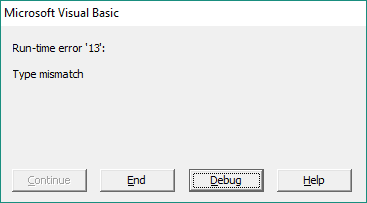Introduction | Example | Tutorial | Applications

## Introduction - VBA Absolute Value

This VBA tutorial shows you how to take the absolute value of a number with the VBA Abs function. The VBA Abs function will return the absolute value of a number, variable or formula in your macro. The VBA Abs function accepts one argument - a number - and will return the magnitude of that number by ignoring its sign.

Keep exercising your macro skills and you too can develop VBA Abs! See what I did there?

Okay, okay. Let’s look at an example.

## Example - VBA Absolute Value

### VBA Abs Function

``````Sub VBA_Absolute_Value()
Dim d1 As Double
Dim d2 As Double
Dim d3 As Double

d1 = 1.5
d2 = -0.8

d3 = Abs(d1 * d2)
Debug.Print d3 'Yields +1.2
End Sub``````

Make powerful macros with our free VBA Developer Kit

Tutorials like this can be complicated. That’s why we created our free VBA Developer Kit and our Big Book of Excel VBA Macros to supplement this tutorial. Grab them below and you’ll be writing powerful macros in no time.

I'll take a free VBA Developer Kit

## Tutorial - VBA Absolute Value

### How to find the absolute value in VBA

First, a refresher on what the absolute value of a number is. The absolute value of a number is a mathematical term meaning the magnitude of a number, ignoring the sign. In other words, the absolute value of a number is always the positive equivalent of the number representing how for away that number is from zero.

The VBA Abs function strips the sign from your numbers or numerical expressions to return the absolute value. To do that, the Abs function accepts one argument: a number. The number can be a variable or even a formula that produces a numeric result. In other words, any numeric expression will do. Here’s the syntax for the Abs function:

`Abs(Number)`

In the example above, the “Number” argument is the product of the variables d1 and d2. If you didn’t take the absolute value, multiplying these variables would produce a -1.2, but the Abs function returns a +1.2 since the sign is ignored.

### Common Error with the Abs function

If you accidentally pass the Abs function a string or other non-numeric value, you will get a “Run-time error ‘13’ Type Mismatch” error.With that said, the Abs function isn’t dumb. If you try to take the absolute value of a string that represents a number, it will properly process the string as a number and return the absolute value. Here’s an example to demonstrate what I mean:

``````Sub VBA_Abs_String()
Debug.Print Abs("-5.4") 'Yields +5.4
End Sub``````

## Application Ideas

You’ll want to use the VBA Abs function anytime you need to absolute value of a number. It’s a good function to use when you’re passing an argument to the VBA Sqr function since you can’t take the square root of a negative number.

That’s all for this tutorial. When you’re ready to take your VBA to the next level, subscribe using the form below.